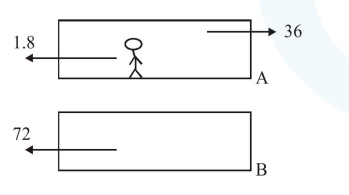# Train A and train B are running on parallel tracks in the opposite directions with speeds

Question:

Train A and train B are running on parallel tracks in the opposite directions with speeds of $36 \mathrm{~km} /$ hour and $72 \mathrm{~km} /$ hour, respectively. A person is walking in train $\mathrm{A}$ in the direction opposite to its motion with a speed of $1.8 \mathrm{~km} / \mathrm{hr}$. Speed (in $\mathrm{ms}^{-1}$ ) of this person as observed from train B will be close to : (take the distance between the tracks as negligible)

1. $30.5 \mathrm{~ms}^{-1}$

2. $29.5 \mathrm{~ms}^{-1}$

3. $31.5 \mathrm{~ms}^{-1}$

4. $28.5 \mathrm{~ms}^{-1}$

Correct Option: , 2

Solution:Velocity of man with respect to ground

$\overrightarrow{\mathrm{V}}_{\mathrm{m} / \mathrm{g}}=\overrightarrow{\mathrm{V}}_{\mathrm{m} / \mathrm{A}}+\overrightarrow{\mathrm{V}}_{\mathrm{A}}=-1.8+36$

Velocity of man w.r.t. B

$\overrightarrow{\mathrm{V}}_{\mathrm{m} / \mathrm{B}}=\overrightarrow{\mathrm{V}}_{\mathrm{m}}-\overrightarrow{\mathrm{V}}_{\mathrm{B}}$

$=-1.8+36-(-72)$

$=106.2 \mathrm{~km} / \mathrm{hr}$

$=29.5 \mathrm{~m} / \mathrm{s}$